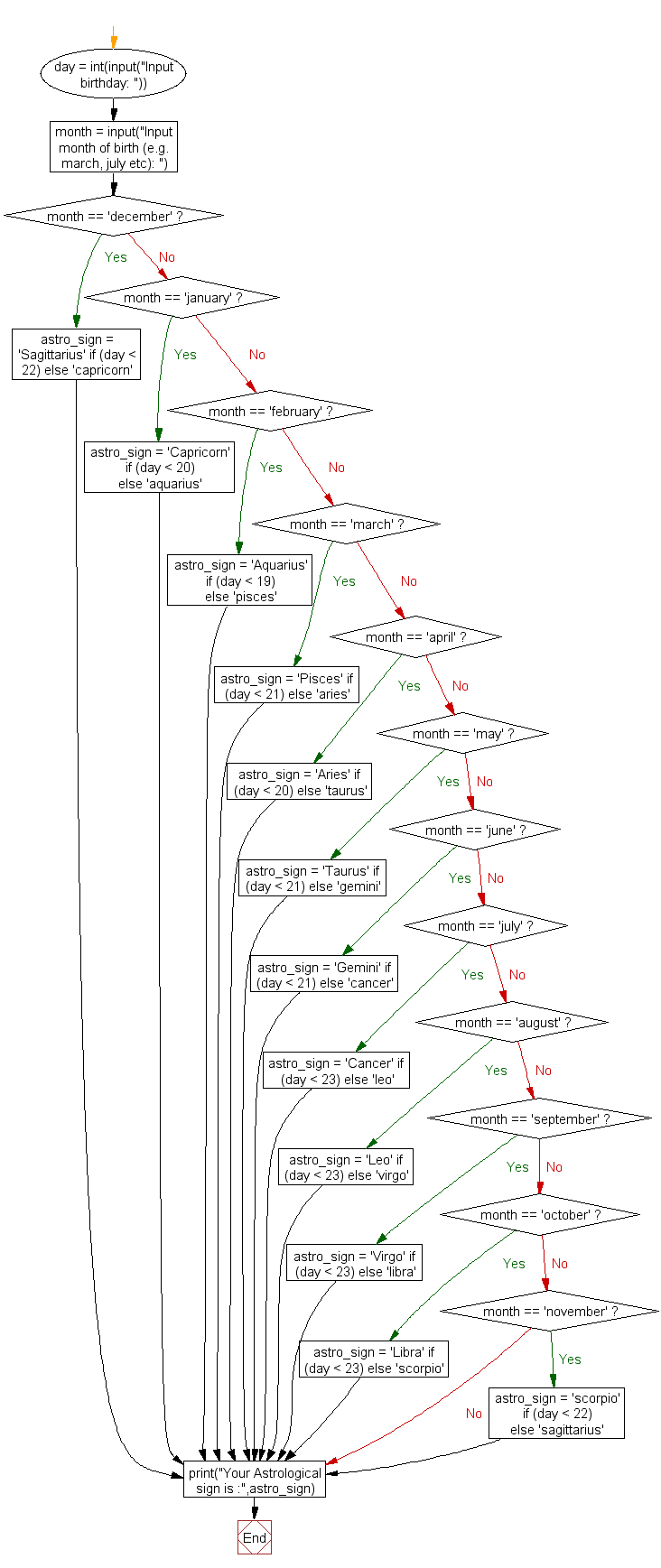﻿ Python Exercise: Display astrological sign for given date of birth - w3resource# Python Exercise: Display astrological sign for given date of birth

## Python Conditional: Exercise - 38 with Solution

Write a Python program to display astrological sign for given date of birth.

Sample Solution:

Python Code:

``````day = int(input("Input birthday: "))
month = input("Input month of birth (e.g. march, july etc): ")
if month == 'december':
astro_sign = 'Sagittarius' if (day < 22) else 'capricorn'
elif month == 'january':
astro_sign = 'Capricorn' if (day < 20) else 'aquarius'
elif month == 'february':
astro_sign = 'Aquarius' if (day < 19) else 'pisces'
elif month == 'march':
astro_sign = 'Pisces' if (day < 21) else 'aries'
elif month == 'april':
astro_sign = 'Aries' if (day < 20) else 'taurus'
elif month == 'may':
astro_sign = 'Taurus' if (day < 21) else 'gemini'
elif month == 'june':
astro_sign = 'Gemini' if (day < 21) else 'cancer'
elif month == 'july':
astro_sign = 'Cancer' if (day < 23) else 'leo'
elif month == 'august':
astro_sign = 'Leo' if (day < 23) else 'virgo'
elif month == 'september':
astro_sign = 'Virgo' if (day < 23) else 'libra'
elif month == 'october':
astro_sign = 'Libra' if (day < 23) else 'scorpio'
elif month == 'november':
astro_sign = 'scorpio' if (day < 22) else 'sagittarius'
```
```

Sample Output:

```Input birthday: 18
Input month of birth (e.g. march, july etc): january
Your Astrological sign is : Capricorn
```

Flowchart :## Visualize Python code execution:

The following tool visualize what the computer is doing step-by-step as it executes the said program:

Python Code Editor:

Have another way to solve this solution? Contribute your code (and comments) through Disqus.

What is the difficulty level of this exercise?

Test your Python skills with w3resource's quiz

﻿

## Python: Tips of the Day

Returns a list with n elements removed from the beginning

Example:

```def tips_take(itr, n = 1):
return itr[:n]
print(tips_take([1, 2, 3], 5))
print(tips_take([1, 2, 3], 0))
```

Output:

```[1, 2, 3]
[]
```